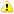# CONVERT Function for Numbers - SQL Server to MySQL Migration

In SQL Server you can use CONVERT function to convert an expression to a number and also use it to truncate or round numeric value.

In MySQL you can also use CONVERT function but note that the order of parameters is different.Also MySQL CONVERT does not support NUMERIC as type, you have to use DECIMAL.SQL Server:

```  -- Convert string to number
SELECT CONVERT(NUMERIC, '1');
# 1
SELECT CONVERT(DECIMAL, '3');
# 3
```

MySQL:

```  -- NUMERIC type is not supported
SELECT CONVERT('1', NUMERIC);
# ERROR 1064 (42000): ... syntax to use near 'NUMERIC)' at line 1

-- DECIMAL is supported, but the parameter order is different
SELECT CONVERT('3', DECIMAL);
# 3```

## Truncating or Rounding Numeric Values

Additionally CONVERT allows you to truncate or round values:

SQL Server:

```  -- Truncate to integer
SELECT CONVERT(NUMERIC, 1.17);
# 1

-- Round to number with single decimal digit
SELECT CONVERT(NUMERIC(3,1), 1.17);
# 1.2```

MySQL:

```  -- Truncate to integer
SELECT CONVERT(1.17, DECIMAL);
# 1

-- Round to number with single decimal digit
SELECT CONVERT(1.17, DECIMAL(3,1));
# 1.2```

For more details, see SQL Server to MySQL Migration.